## Logic 1

Recall that in Engr 121 we used arithmetic operations instead of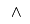,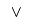and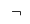1. What was the symbol for each?should be natural, the others a little forced
2. Draw a truth table for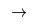and determine which arithmetic operation is appropriate An inequality

## Logic 2

On first glance, the statements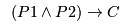and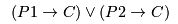appear to say different things.
1. Compute a truth table for both. What is the result?
2. Similarly, for each statement, find all situations where it is false. What do you see here?
3. Rewrite both statements using the law of contrapositive. Is this clearer?
4. Directly prove that ifis true, thenis true. You might need cases Assume either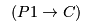or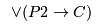is true .....
5. It is difficult to prove the converse statement, if, then. Give it a go! Focus on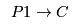, consider the possibilities Eitheris true, or it is false. One case is easy ..

## Quantifiers

1. Explain why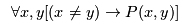allows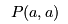to be true.
2. What does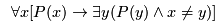say?
3. What is the negation of the previous statement?

## Induction

1. This is a statement of what induction does, the set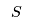is the collection of natural numbers collected by the process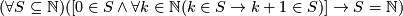This is very complicated!
1. Which part is the base case?
2. Which part is the induction hypothesis and step?
3. The last part is a promise, what is the promise?
4. What is the negation of the statement and what does it say?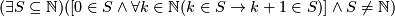There is some subset of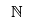for which induction doesn't work, it misses some number.
2. The least number principle (which we haven't seen) says that every non-empty subset ofhas a least element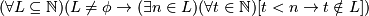1. Which part talks about the set being non-empty?
2. What is the least element?
3. Negate this statement and explain what is says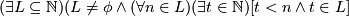There is a subset of, no matter where we think the bottom of the set is, we can always go lower.
4. Use induction to prove that the least number principle is true.
Look at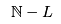, the set of values that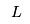doesn't collect
Do induction on, you should find that it satisfies the conditions, so it collects everything ...